Next: Geometric Transformations Up: Computer Vision IT412 Previous: Spatial domain methods

Subsections

# Frequency domain methods

Image enhancement in the frequency domain is straightforward. We simply compute the Fourier transform of the image to be enhanced, multiply the result by a filter (rather than convolve in the spatial domain), and take the inverse transform to produce the enhanced image.

The idea of blurring an image by reducing its high frequency components, or sharpening an image by increasing the magnitude of its high frequency components is intuitively easy to understand. However, computationally, it is often more efficient to implement these operations as convolutions by small spatial filters in the spatial domain. Understanding frequency domain concepts is important, and leads to enhancement techniques that might not have been thought of by restricting attention to the spatial domain.

## Filtering

Low pass filtering involves the elimination of the high frequency components in the image. It results in blurring of the image (and thus a reduction in sharp transitions associated with noise). An ideal low pass filter would retain all the low frequency components, and eliminate all the high frequency components. However, ideal filters suffer from two problems: blurring and ringing. These problems are caused by the shape of the associated spatial domain filter, which has a large number of undulations. Smoother transitions in the frequency domain filter, such as the Butterworth filter, achieve much better results.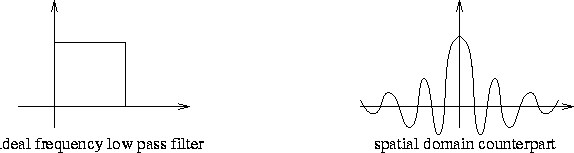## Homomorphic filtering

Images normally consist of light reflected from objects. The basic nature of the image F(x,y) may be characterized by two components: (1) the amount of source light incident on the scene being viewed, and (2) the amount of light reflected by the objects in the scene. These portions of light are called the illumination and reflectance components, and are denoted i(x,y) and r(x,y) respectively. The functions i and r combine multiplicatively to give the image function F:

F(x,y) = i(x,y)r(x,y),

where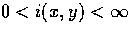and 0 < r(x,y) < 1. We cannot easily use the above product to operate separately on the frequency components of illumination and reflection because the Fourier transform of the product of two functions is not separable; that isSuppose, however, that we defineThenor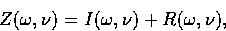where Z, I and R are the Fourier transforms of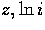and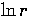respectively. The function Z represents the Fourier transform of the sum of two images: a low frequency illumination image and a high frequency reflectance image.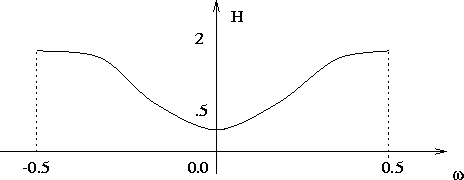If we now apply a filter with a transfer function that suppresses low frequency components and enhances high frequency components, then we can suppress the illumination component and enhance the reflectance component. Thus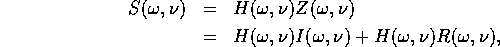where S is the Fourier transform of the result. In the spatial domain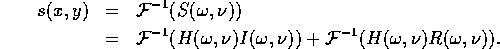By lettingand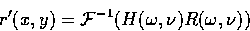we get

s(x,y) = i'(x,y) + r'(x,y).

Finally, as z was obtained by taking the logarithm of the original image F, the inverse yields the desired enhanced image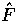: that is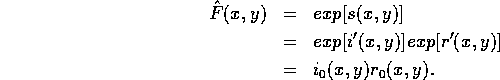Thus, the process of homomorphic filtering can be summarized by the following diagram: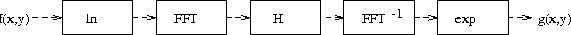Next: Geometric Transformations Up: Computer Vision IT412 Previous: Spatial domain methods
Robyn Owens
10/29/1997